Courses

# Doc: Colligative Properties of Solutions Notes | EduRev

## Chemistry Class 12

Created by: Mohit Rajpoot

## Class 12 : Doc: Colligative Properties of Solutions Notes | EduRev

The document Doc: Colligative Properties of Solutions Notes | EduRev is a part of the Class 12 Course Chemistry Class 12.
All you need of Class 12 at this link: Class 12

COLLIGATIVE PROPERTIES
A dilute solution is one in which the amount of the solute is very small in comparison to the amount of the solvent. The dilute solutions show more or less ideal behavior as the heat and volume changes, accompanying the mixing of solute and solvent, are negligible for all practical purposes. Dilute solutions obey

Raoult’s law. The properties of dilute solutions which depend only on number particles of solute present in the solution and not on their identity are called colligative properties (denoting depending upon collection).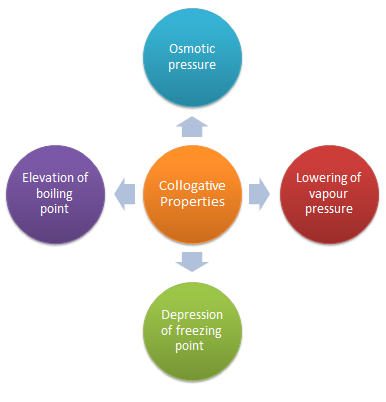We shall assume here that the solute is non volatile, so it does not contribute to the vapour. We shall also assume that the solute does not dissolve in solid solvent, that is, the pure solid solvent separates when the solution is frozen, the latter assumption is quite drastic, although it is true for many mixtures, it can be avoided.

Colligative properties are the properties of only dilute solution which are supposed to behave as ideal solutions. The various colligative properties are:
Depression of freezing point

• Lowering of vapour pressure
• Osmotic pressure
• Elevation of boiling point

Lowering of Vapour Pressure by Non Volatile Solute
It has been known for a long time that when a non-volatile solute is dissolved in a liquid, the vapour pressure of the solution becomes lower than the vapour pressure of the pure solvent. In 1886, the French chemist, Francois Raoult, after a series of experiments on a number of solvents including water, benzene and ether, succeeded in establishing a relationship between the lowering of vapour pressure of a solution and the mole fraction of the non-volatile solute.
Let us consider a solution obtained by dissolving n moles of a non-volatile solute in N moles of a volatile solvent. Then mole fraction of the solvent, X1 = N/(n + N) and mole fraction of the solute, X2 = n /(N + n). Since  the solute is non-volatile, it would have negligible vapour pressure. The vapour pressure of the solution is, therefore merely the vapour pressure of the solvent. According to Raoult's law, the vapour pressure of a solvent (P1) in an ideal solution is given by the expression;
P1 = X1 P01       ...(1)
where P01 is the vapour pressure of the pure solvent. Since X1 + X2 = 1, Eq. 1 may be written as
P1 = (1 - X2) P01      ...(2)
or P1/P01 = 1 - X2
or P1 - P01/P01 = X2    ...(3)
The expression on the left hand side of Equation (3) is usually called the relative lowering of vapour pressure. Equation (3) may thus be stated as: `The relative lowering of vapour pressure of a solution containing a non-volatile solute is equal to the mole fraction of the solute present in the solution.'
This is one of the statements of the Raoult's law.
Since mole fraction of the solute, X2 is given by n/(N + n), Equation (3) may be expressed as
P01 - P1/P01 = n/N + n  ....(4)
It is evident from Equation (4) that the lowering of vapour pressure of a solution depends upon the number of moles (and hence on the number of molecules) of the solute and not upon the nature of the solute dissolved in a given amount of the solvent. Hence, lowering of vapour pressure is a colligative property.

Determination of Molar Masses from Lowering of Vapour Pressure
It is possible to calculate molar masses of non-volatile non-electrolytic solutes by measuring vapour pressures of their dilute solutions.
Suppose, a given mass, w gram, of a solute of molar mass m, dissolved in
W gram of solvent of molar mass M, lowers the vapour pressure from P01 to P1.

Then , by Equation  (4)Limitations of Raoult’s Law

• Raoult’s law is applicable only to very dilute solutions.
• It is applicable to solutions containing non-volatile solute only.
• It is not applicable to solutes which dissociate or associate in a particular solution.

Example 1. The density of a 0.438 M solution of potassium chromate at 298 K is 1.063 g cm-3. Calculate the vapour pressure of water above this solution. Given : P0 (water) = 23.79 mm Hg.
Solution.

A solution of 0.438 M means 0.438 mol of K2CrO4 is present in 1L of the solution. Now,
Mass of K2CrO4 dissolved per litre of the solution  = 0.438 × 194  = 84.972 g
Mass of 1L of solution = 1000 × 1.063 = 1063 g
Amount of water in 1L of solution = 978.028/18  = 54.255 mol
Assuming K2CrO4 to be completely dissociated in the solution, we will have;
Amount of total solute species in the solution = 3 × 0.438 = 1.314 mol.
Mole fraction of water solution = 54.335/(54.335 + 1.314) = 0.976
Finally, Vapour pressure of water above solution = 0.976 × 23.79  = 23.22 mm Hg

Boiling Point Elevation by a Non-Volatile Solute
The boiling point of a liquid is the temperature at which its vapour pressure becomes equal to 760 mm (i.e. 1 atmospheric pressure). Since the addition of a non-volatile solute lowers the vapour pressure of the solvent, the vapour pressure of a solution is always lower than that of the pure solvent, and hence it must be heated to a higher temperature to make its vapour pressure equal to atmospheric pressure. Thus the solution boils at a higher temperature than the pure solvent. If Tb0 is the boiling point of the solvent and Tb is the boiling point of the solution, the difference in boiling points ( δ  Tb) is called the elevation of boiling point.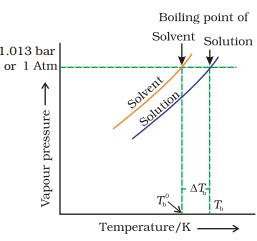Thus, Tb – T0b  = δ Tb.
δ Ta molality where  δ Tb = elevation of boiling point
n= no. of moles of non-volatile solute
N= Total no. of moles in the solution
This implies that the boiling point elevation in a dilute solution is directly proportional to the number of moles of the solute dissolved in a given amount of the solvent and is quite independent of the nature of the solute. Hence, boiling point elevation is a colligative property
δ Tb = Kb*m
kb :  molal elevation constant or Ebullioscopic constant
m :   molality of the solution

Molal boiling point elevation constant or ebullioscopic constant of the solvent, is defined as the elevation in boiling point which may theoretically be produced by dissolving one mole of any solute in 1000 g of the  solvent.
or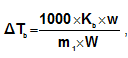where m1 = molecular weight of solute and w and W are weights of solute and solvent.

Depression of Freezing Point by a Non-Volatile Solute
Freezing point is the temperature at which solid and liquid states of a substance have the same vapour pressure. It is observed that the freezing point of the solution (Tf) containing non volatile solute is always less than the freezing point of the pure solvent (Tf0). Thus,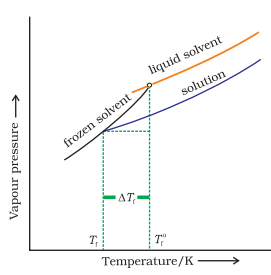T0f - Tf =  δ Tf
It can be seen that
δ Tf  α molality
that, is freezing point depression of a dilute solution is directly proportional to the number of moles of the solute dissolved in a given amount of the solvent and is independent of the nature of solute
or    δ T= Kfm
kf : molal freezing point depression constant of the solvent or cryoscopic constant
m : molality of the solution
Molal freezing point depression constant of the solvent or cryoscopic constant, is defined as the depression in freezing point which may theoretically be produced by dissolving 1 mole of any solute in 1000g of the solvent.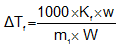where m1 = molecular weight of solute and w and W are weights of solute and solvent.

Offer running on EduRev: Apply code STAYHOME200 to get INR 200 off on our premium plan EduRev Infinity!

,

,

,

,

,

,

,

,

,

,

,

,

,

,

,

,

,

,

,

,

,

;